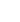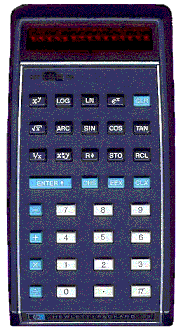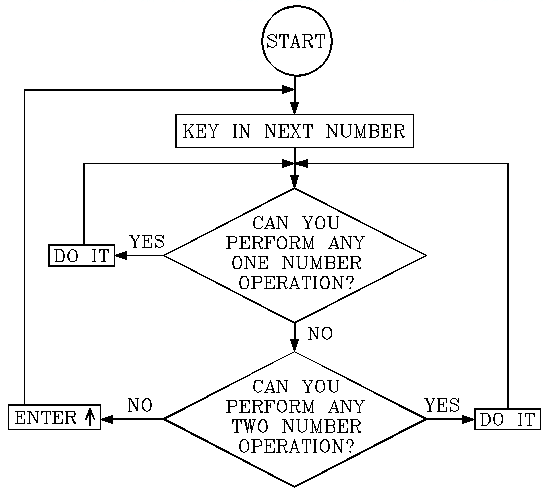W. Marshall Leach, Jr.
Professor of Electrical and Computer Engineering
Georgia Institute of Technology
Atlanta, Georgia 30332-0250The operating system for Hewlett Packard scientific calculators is called "reverse Polish notation," or simply rpn. In talking to students who have HP calculators, I have not found one who knows the rpn algorithm. This is unfortunate, because not knowing it is a major cause for making mistakes with HP calculators. This page explains the algorithm and gives examples. When the algorithm is applied correctly, one can use the calculator to rapidly evaluate long expressions without stopping to think how the terms are grouped in the expression, even with the calculator display covered.Aside from computer programmers, not many engineers had heard of reverse Polish notation until Hewlett Packard introduced the HP35 calculator in 1972. It was a non-programmable, four-function scientific calculator with only one memory register. It had a light emitting diode display that drew so much current from the batteries that an ac charger came with the calculator. To keep the batteries charged, most people left the calculator connected to the charger when possible. The calculator sold for \$395. In 1973, the price was reduced to \$295. It was discontinued in 1975.The major difference between the HP35 and calculators made by other companies was that it used reverse Polish notation. This was the major feature that HP promoted for the calculator. The HP35 manual had an appendix devoted to explaining the rpn algorithm. As later calculator models were introduced, the emphasis on rpn gradually diminished. The manuals that come with HP calculators today barely even mention it.Polish notation was described in the 1920s by Polish mathematician Jan Lukasiewicz as a logical system for the specification of mathematical equations without parentheses. There are two versions, prefix notation and postfix notation. In prefix notation, the operators are placed before the operand. In postfix notation, this order is reversed. The following example illustrates the two. The asterisk is used for the multiplication sign.

```   Equation with parenthesis       (1 + 2) * 3
Prefix notation                 * 3 + 1 2       or      * + 1 2 3
Postfix notation                1 2 + 3 *       or      3 1 2 + *
```

Postfix notation has since become known as reverse Polish notation. In the HP implementation of rpn, the ENTER key is pressed between any two numbers that are not separated by an operation.

### The AlgorithmThe basic reverse Polish calculator algorithm is to key in a number. If you can perform a calculator operation do it. If not, press ENTER. Then repeat until the complete expression is evaluated. The following flow graph summarizes the algorithm.### ExamplesIf you own one of the HP calculators that allows you to set the display in either the symbolic mode or the numeric mode, set the mode to numeric. This is done by entering the mode/flag menu and flagging the line that says "constants to symbols" so that it says "constants to numbers." Otherwise, you will not obtain numerical answers when you use symbols such as "pi" in equations.There are two evaluation methods given for each example. The first evaluates the expression in the order that the numbers appear. The second evaluates an equivalent expression in which the order of some terms is reversed so as to minimize the number of times the ENTER key is pressed. This minimizes the chances of overflowing the calculator stack for the models that have only four stack registers. Letters for calculator functions are upper case, * is used for the multiplication key, / is used for the divide key, e^x is used for the "e to the x" key, x^2 is used for the "x squared" key, and ROOT(x) is used for the "square root of x" key. The columns to the right show the contents of the X, Y, Z, and T registers after each operation. Note how the numbers move to the right when ENTER is pressed and to the left after an operation is performed.

Example 1:

```   Algebraic Expression (4 + 2 * 5) / (1 + 3 * 2)

Reverse Polish Expression 4 2 5 * + 1 3 2 * + /

HP Calculator Implementation

X	Y	Z	T
4		4	.	.	.
ENTER		4	4	.	.
2		2	4	.	.
ENTER		2	2	4	.
5		5	2	4	.
*		10	4	.	.
+		14	.	.	.
1		1	14	.	.
ENTER		1	1	14	.
3		3	1	14	.
ENTER		3	3	1	14
2		2	3	1	14
*		6	1	14	.
+		7	14	.	.
/		2	.	.	.

Reordered Algebraic Expression (2 * 5 + 4 )/(3 * 2 + 1)

Reverse Polish Expression 2 5 * 4 + 3 2 * 1 + /

HP Calculator Implementation

X	Y	Z	T
2		2	.	.	.
ENTER		2	2	.	.
5		5	2	.	.
*		10	.	.	.
4		4	10	.	.
+		14	.	.	.
3		3	14	.	.
ENTER		3	3	14	.
2		2	3	14	.
*		6	14	.	.
1		1	6	14	.
+		7	14	.	.
/		2	.	.	.

The answer is 2 for both methods. Note that the numbers never
reach the T register in Method 2.
```Each time ENTER is pressed, the numbers move up in the stack. Each time an operation is performed, the numbers move down in the stack. Some HP calculator models have only 4 registers and it is possible to overflow the stack if ENTER is pressed too many times without operations in between. For this reason, Method 2 is preferred where products are evaluated before sums. Note that Method 2 contains 2 less ENTERs and the numbers never reach the T register. It should be obvious that the number of ENTERs can be minimized if the expression is rearranged so that multiplications and divisions are performed before additions and subtractions. The rearrangement can easily be done mentally without rewriting the expression.

Example 2:

```   Algebraic Expression [5 + 8 * sin(2 * 15)] / [2 + tan(45)]

Reverse Polish Expression 5 8 2 15 * sin * + 2 45 tan + /

HP Calculator Implementation

X	Y	Z	T
5		5	.	.	.
ENTER		5	5	.	.
8		8	5	.	.
ENTER		8	8	5	.
2		2	8	5	.
ENTER		2	2	8	5
15		15	2	8	5
*		30	8	5	.
SIN		0.5	8	5	.
*		4	5	.	.
+		9	.	.	.
2		2  	9	.	.
ENTER		2	2	9	.
45		45	2	9	.
TAN		1	2	9	.
+		3	9	.	.
/		3	.	.	.

Reordered Algebraic Expression [sin(2 * 15) * 8 + 5] / [tan(45) + 2]

Reverse Polish Expression 2 15 * sin 8 * 5 + 45 tan 2 + /

HP Calculator Impelementation

X	Y	Z	T
2		2	.	.	.
ENTER		2	2	.	.
15		15	2	.	.
*		30	.	.	.
SIN		0.5	.	.	.
8		8	0.5	.	.
*		4	.	.	.
5		5	4	.	.
+		9	.	.	.
45		45	9	.	.
TAN		1	9	.	.
2		2	1	9	.
+		3	9	.	.
/		3	.	.	.

The answer is 3 for both methods.
```Method 2 requires 3 less ENTERs.

Example 3:

```   Algebraic Expression [3 * ln(e^2) + 8 * cos(60)] / [3 * 4^0.5 - 1]

Reverse Polish Expression 3 e ^2 ln * 8 60 cos * + 3 4 ^0.5 * 1 - /

HP Calculator Implementation

X	Y	Z	T
3               3       .       .       .
ENTER           3       3       .       .
1               1       3       .       .
e^x             2.718   3       .       .
x^2             7.389   3       .       .
LN              2       3       .       .
*               6       .       .       .
8               8       6       .       .
ENTER           8       8       6       .
60              60      8       6       .
COS             0.5     8       6       .
*               4       6       .       .
+               10      .       .       .
3               3       10      .       .
ENTER           3       3       10      .
4               4       3       10      .
ROOT(x)         2       10      .       .
*               6       10      .       .
1               1       6       10      .
-               5       10      .       .
/               2       .       .       .

Reordered Algebraic [ln(e^2) * 3 + cos(60) * 8] / [4^0.5 * 3 - 1]

Reverse Polish e ^2 ln 3 * 60 cos 8 * + 4 ^0.5 3 * 1 - /

HP Calculator Implementation

X	Y	Z	T
1                1       .       .       .
e^x              2.718   .       .       .
x^2              7.389   .       .       .
LN               2       .       .       .
3                3       2       .       .
*                6       .       .       .
60               60      6       .       .
COS              0.5     6       .       .
8                8       0.5     6       .
*                4       6       .       .
+                10      .       .       .
4                4       10      .       .
ROOT(x)          2       10      .       .
3                3       2       10      .
*                6       10      .       .
1                1       6       10      .
-                5       10      .       .
/                2       .       .       .

The answer is 2 for both methods.
```With a little practice, it is possible to rapidly evaluate long expressions containing many nested parentheses without ever stopping to think about how terms are grouped. Always start in the innermost parentheses and work out, evaluating products and quotients before sums and differences. Once you get the hang of it, you can even do complex calculations with the display window covered and get the right answers. Try that with a calculator that uses algebraic notation, and chances are you will get a different answer every time if you don't get lost first. That tends to happen to me with algebraic notation calculators even with the display window uncovered. If you own a HP calculator and think in terms of algebraic notation, go buy a TI calculator and forget this page.

Home.This page is not a publication of the Georgia Institute of Technology and the Georgia Institute of Technology has not edited or examined the content. The author of this page is solely responsible for the content.